# Quiz 13: Measuring and Evaluating Financial Performance

Business

1. Calculate Gross Profit Margin Ratio: Gross profit margin ratio indicates the relation between sales and gross profit. It measures the percentage of gross profit generated by each amount of sales. Gross profit margin ratio will be calculated by using following formula.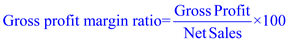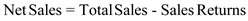Calculate Gross Profit for both the years: Substitute \$15,000 for Gross profit and \$80,000 for Net sale.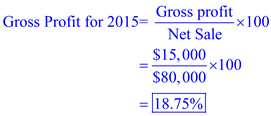Therefore, Gross profit for 2015 is 18.75%. Substitute \$8,000 for Gross profit and \$56,000 for Net sale.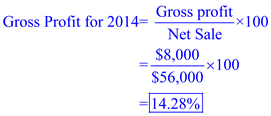Therefore, Gross profit for 2014 is 14.28%. Calculate Net Profit Margin Ratio: Net profit margin ratio indicates the relation between sales and net profit. It measures the percentage of net profit generated by each amount of sales. Net profit margin ratio will be calculated by using following formula.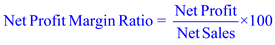Calculate Net Profit for both the years: Substitute \$6,950 for Net profit and \$80,000 for Net sales.Therefore, Net profit for 2015 is 8.68% Substitute \$2,100 for Net profit and \$56,000 for Net sales.Therefore, Net profit for 2015 is 8.68% The net profit margin increased and therefore is going in the right direction. Calculate Return on equity ratio: Return on equity ratio is calculated to find out how efficiently the fund supplied by the equity shareholders has been used. It is calculated as follows: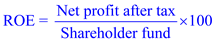Calculate Return on equity for both the years: Substiute \$6,950 for Net profit after tax and \$31,950 for shareholder fund.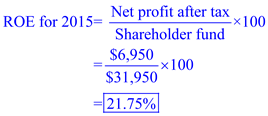Therefore, return on equity for 2015 is 21.75%. Substiute \$2,100 for Net profit after tax and \$32,100 for shareholder fund.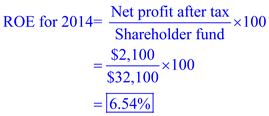Therefore, return on equity for 2015 is 21.75%. These results show that implies the trend is going in the right direction. The company is earning high gross profit from each dollar of sales. 2. Calculate Current ratio: Current ratio: Current ratio shows the company ability to repay their short term obligations like accounts payable, salaries payable, dividend payable, etc. Users can understand easily company's liquidity by using this current ratio. A higher current ratio indicates higher liquidity position of the company, and lower ratio indicates less liquidity position. Current ratio will be calculated by using following formula.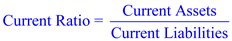Calculate Net Profit for both the years: Substitute \$ 10,950 for Current assets and \$8,000 for current liabilities.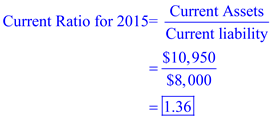Therefore, Current ratio for 2015 is 1.36. Substitute \$ 11,100 for Current assets and \$8,000 for current liabilities.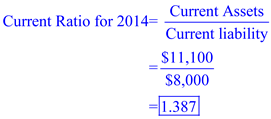Therefore, Current ratio for 2015 is 1.387 From the above calculation we can say that in 2015 company will be more liquid. 3. Calculate Debt to equity ratio: Debt to equity ratio shows the relationship between shareholder equity and total debt of the firm to identify the leverage capacity of the company. It is calculated as follows: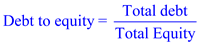Calculate Net Profit for both the years: Substitute \$43,000 for Total debt and \$74,950 for Total equity.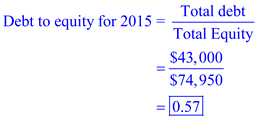Therefore, Debt to equity for 2015 is 0.57. Substitute \$58,000 for Total debt and \$90,100 for Total equity.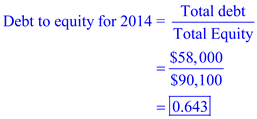Therefore, Debt to equity ratio for 2014 is 0.643. Time interest earned ratio: Time interest earned ratio indicates the relationship between net profit before tax and interest on long term debt. It determines the debt servicing capacity of a business enterprise keeping in view fixed interest on long term debt. Calculate Time interest earned ratio by using following formula.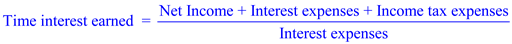Calculate Time interest earned ratio for both the years: Substitute \$6,950 for Net income, \$1,000 for interest expenses, \$3,050 for income tax expenses.Therefore, Time interest earned ratio for 2015 is 11. Substitute \$2,100 for Net income, \$3,100 for interest expenses, \$800 for income tax expenses.Therefore, Time interest earned ratio for 2015 is 11. From the above calculation we can say that in 2015 company will be more solvent.

1. Horizontal analysis: Horizontal analysis is a comparison between two years financial statements. This is done by taking one year as base year. All the items in a year will be compared with same item of the base year, and result will be shown in percentage. Financial statement users can easily understand performance of the company by using this horizontal analysis. Prepare horizontal analysis income statement for the year ended December 31: Calculations required doing horizontal analysis for both the year will be as follows: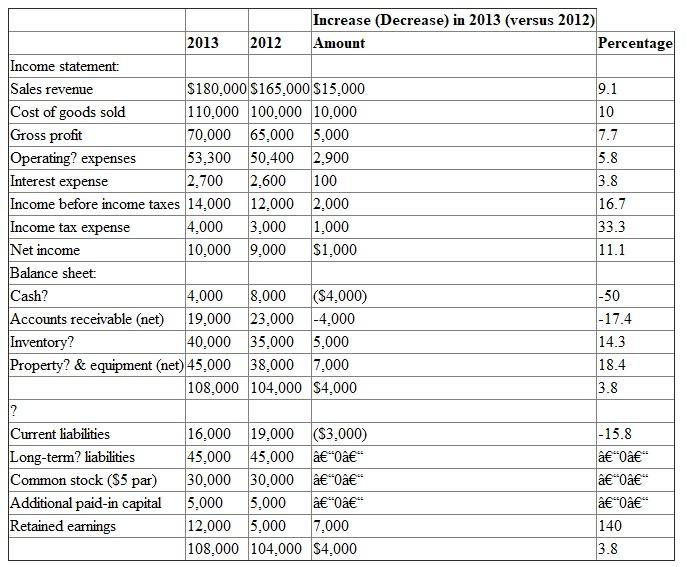Working Note: Take 2012 as base year and calculate the percentages. Below shown how to calculate percentage. Sales revenue percentage:Sales revenue percentage is 9.1%. Therefore, calculate all percentages like that. 2. (a) The total percent change taken place in Cash is high but it is due to small dollar balance. The total percent change in Retained Earnings is also big; it is also due to small dollar balance. (b) One unusual change is that although sales increased by 9.1%, accounts receivable decreased by 17.4%. Typically, an increase in sales would be accompanied by an increase in accounts receivable.

1) Prepare horizontal analysis: Horizontal analysis is a comparison between two years financial statements. This is done by taking one year as base year. All the items in a year will be compared with same item of the base year, and result will be shown in percentage. Financial statement users can easily understand performance of the company by using this horizontal analysis. Calculations required doing horizontal analysis for both the year will be as follows: Change in dollars is the difference amount between 2009 and 2010. Change in percentage will calculate through following formula: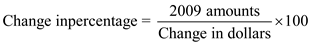2010 and 2009 data is given in the problem.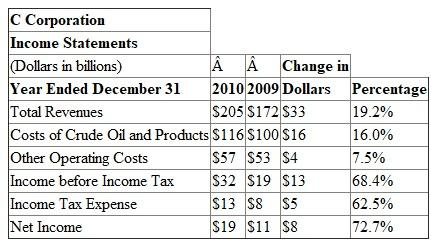• The 18% increase in the average gas price was less than the 19.2% increase in total revenues and more than the 16.0% increase in cost of crude oil and products. • It appears from the analysis that the increase in gas prices explains only part of C's increase in total revenues. 2) Prepare vertical analysis: Vertical analysis is a comparison between two items in a statement. Usually each item of the balance sheet compared with total assets, and each item of the income statement compared with sales revenue of that income statement. Vertical analysis is useful for users to compare percentage of the item for different years. Hence, sales, total assets, and total liabilities equity are taken as 100%. Calculations required doing vertical analysis for both the year will be as follows: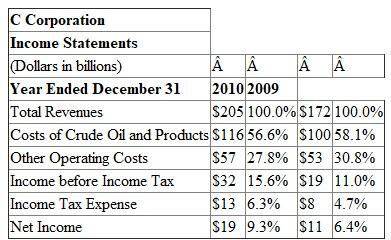• As a percent of total revenues, C's cost of crude oil and products was higher in 2009 (58.1%) than in 2010 (56.6%). • This implies that C Company earned more profit (excluding income tax and other operating costs) per dollar of revenues in 2010 than in 2009.

There is no answer for this question

There is no answer for this question